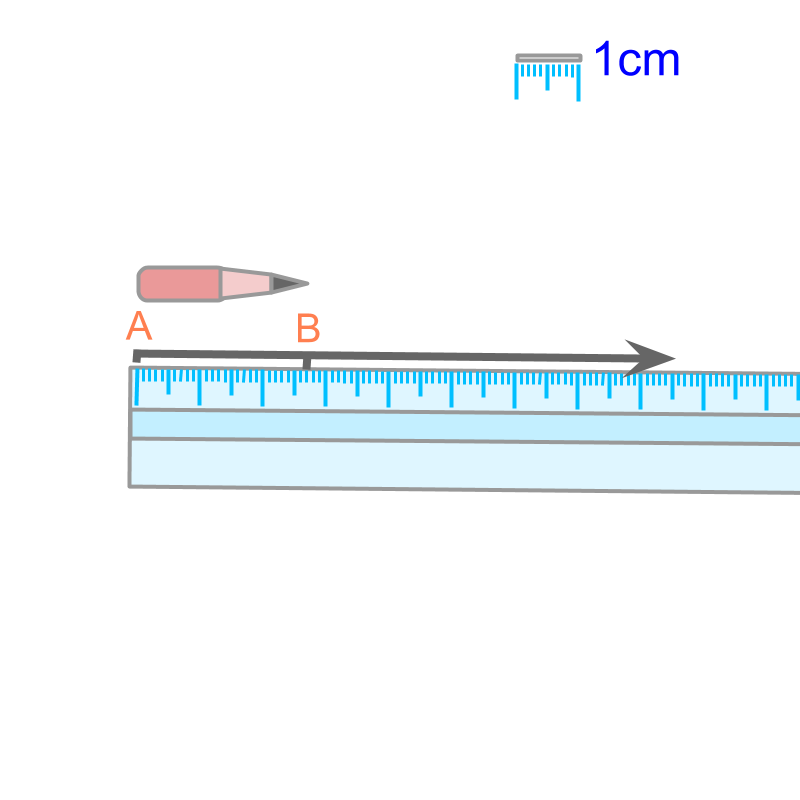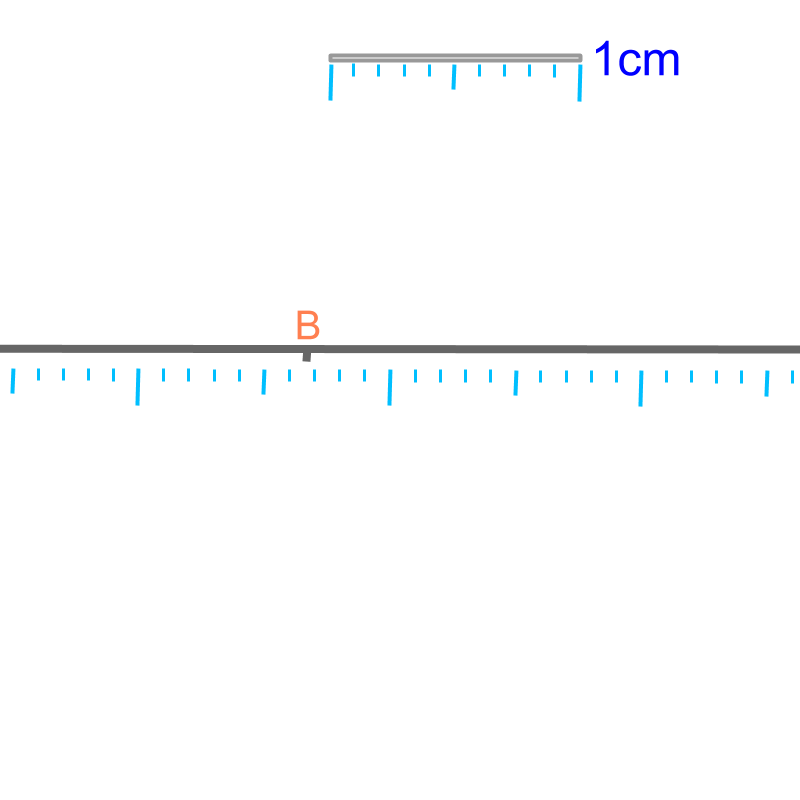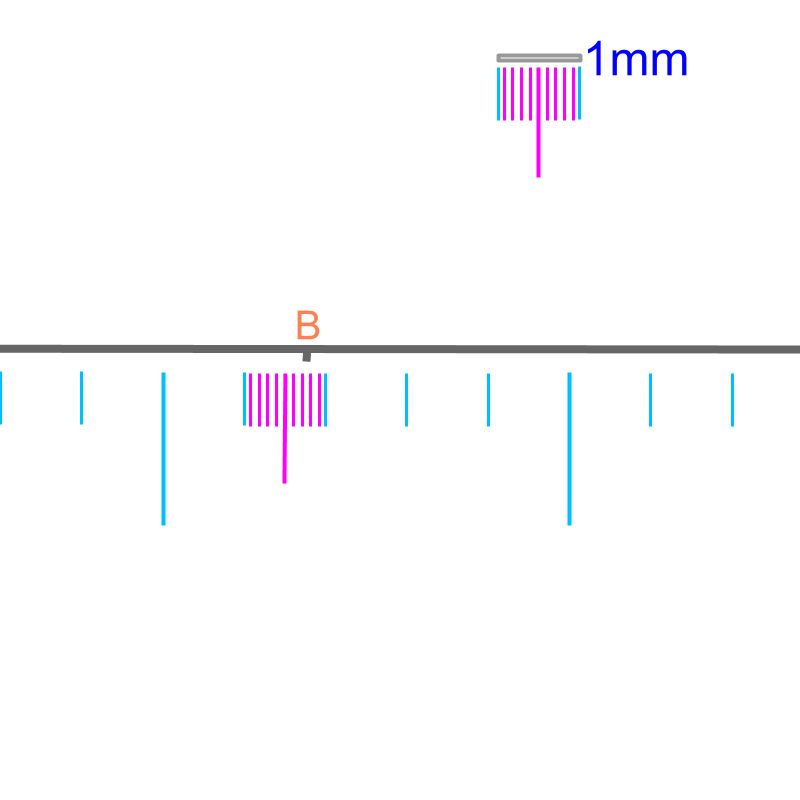maths > decimals

Decimals: Not repeating and not ending

what you'll learn...

overview

On measuring a real-life object, it is found that a number can have decimal digits that do not repeat and do not end. Such decimal numbers -- with decimal digits that do not repeat and do not terminate -- are called "irrational numbers".

numbers in practice

Consider length of a pencil. One of the ways to measure the length of a pencil, is to mark the ends of the pencil into a line-segment and measure the length of the line-segment. This essentially measures the "distance-span between the two ends of the pencil".To measure the length of a pencil, an equivalent line segment is taken. The line segment and the graduated scale are shown in the figure. Note the legend on the right-top of the figure showing $1$$1$ centimeter. What is the length of the line segment $\overline{AB}$$\overline{A B}$?The figure is magnified. Note the legend showing the modified $1cm$$1 c m$ length. The point $A$$A$ is not shown. "the length of $\overline{AB}$$\overline{A B}$ is approximately $2.7cm$$2.7 c m$"The figure is magnified even larger. Note the legend showing the modified $1mm$$1 m m$ length. The length of $\overline{AB}$$\overline{A B}$ is better approximated to $2.68cm$$2.68 c m$

at what accuracy?The line-segment $\overline{AB}$$\overline{A B}$ can be measured at different accuracies.

•  The length is $2.6cm$$2.6 c m$ when measured with a graduated-scale.

•  The length is $2.68cm$$2.68 c m$ when magnified and measured with a better instrument than a scale.

•  The length is $2.68892\cdots$$2.68892 \cdots$ when measured at much higher accuracy. For most practical applications, the length of $2.6cm$$2.6 c m$ is good enough.

Note 1: The line represents a real object. It can be a leaf, or a rod. The important point being, the measurements in real-life are approximated.

Note 2: The length of a real object can be exactly measured too. For example, a pencil of length exactly $2$$2$cm is possible. That object, when magnified and measured with high accuracy, will be of length $2$$2$cm only.

Note 3: The length of a real object can be a decimal number, that does not end or does not repeat. For example, a pencil is of length $3.68023\cdots$$3.68023 \cdots$cm length. The decimals in the number does not end or repeat. It is an interesting observation, that will help to understand some concepts later.

The word "accuracy" means: state of being exactly correct.

defining

On measuring a real-life object, it is found that a number can have decimal digits that do not repeat and do not end. Such decimal numbers -- with decimal digits that do not repeat and do not terminate -- are called "irrational numbers".

The word "irrational" means: that cannot be given as a ratio.

Irrational Numbers : Decimal numbers that has decimal digits that do not end and do not have a pattern of digits that repeats. eg: accurate length of a pencil is $2.68892\cdots$$2.68892 \cdots$.

There are two types of decimal numbers with decimal digits that do not end and do not have a pattern that repeats.

•  Algebraic irrational numbers: Irrational numbers that are solutions to algebraic equations. eg: The square root of $2$$2$ which is a solution to ${x}^{2}=2$${x}^{2} = 2$.

•  Transcendental irrational numbers: Numbers that are not solutions to algebraic equations. The ratio of circumference to diameter of a circle is an irrational numbers. The diameter is chosen to be in the given standard $1$$1$cm. Circle is a regular curve and the length of the curve is measured accurately and found to be an irrational number.

In transcendental irrational numbers, the following is included.
Accurate measurement of real-life object in a unrelated standard.

eg: $1$$1$ pound is approximated to $453.5924277$$453.5924277$ grams. Pound is a standard defined based on mass of $7000$$7000$ grains (wheat or barley). Kilogram is a standard defined based on mass of $1$$1$ liter water at the temperature of melting point. This irrational number is similar to the example illustrated above -- accurately measuring a real-life object.

Similarly, curve-length of a circle is a measurement in an unrelated standard and so is a irrational number.

summary

Irrational Numbers : Decimal numbers that has decimal digits that do not end and do not have a pattern of digits that repeats. eg: accurate length of a pencil is $2.68892\cdots$$2.68892 \cdots$.

Outline